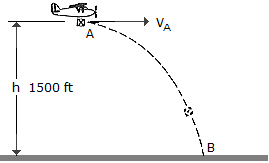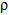# Engineering Mechanics - Kinematics of Particle (KOP) - Discussion

### Discussion :: Kinematics of Particle (KOP) - General Questions (Q.No.5)

5.A package is dropped from the plane which is flying with a constant horizontal velocity of vA = 150 ft/s at a height h = 1500 ft. Determine the radius of curvature of the path of the package just after it is released from plane at A.

 [A].2 = 9860 ft [B].2 = 3000 ft [C].2 = 1500 ft [D].2 = 8510 ft

Explanation:

No answer description available for this question.

 M Kumara Swamy said: (Jun 1, 2016) To find the radius of curvature first you have to find the equation of path in terms of x and y in the cartesian plane: Here x = va * t. y = 0.5 gt^2. Overall y = 0.5(g/v^2) * x^2. Now use formula: Radius of curvature = ([1 + (dy/dx)^2]^1.5)/second order derivative of y. I got 8300 as an answer because I took g = 10 for my convenience of calculation.

 Minam Tungekar said: (Sep 4, 2020) According to me, the solution is; x-direction equation of motion : s = ut + 1/2 at^2 ( u = 150 ft/s, a in x-direction is 0 as the plane is moving with a constant velocity, s = x) x = 150t; t = x/150; y-direction Equation of motion : s = ut + 1/2 at^2 ( here "u" in the y-direction is 0 because as soon as the package is released it will have a velocity tangential to the falling projectile curve it will produce, a is only due to gravitational force g = 32.2 slug/ft^2) y = 1/2 * 32.2 * t^2 ; y = (16.1/22,500) * x^2; y' = (16.1/22,500) * 2x; y'' = (16.1/22,500) * x; The radius of curvature, R at x=0( just after release from plane at point A) = [ [1 + {(y')^2}]^(3/2) / y'']. R = 698.75 ft.